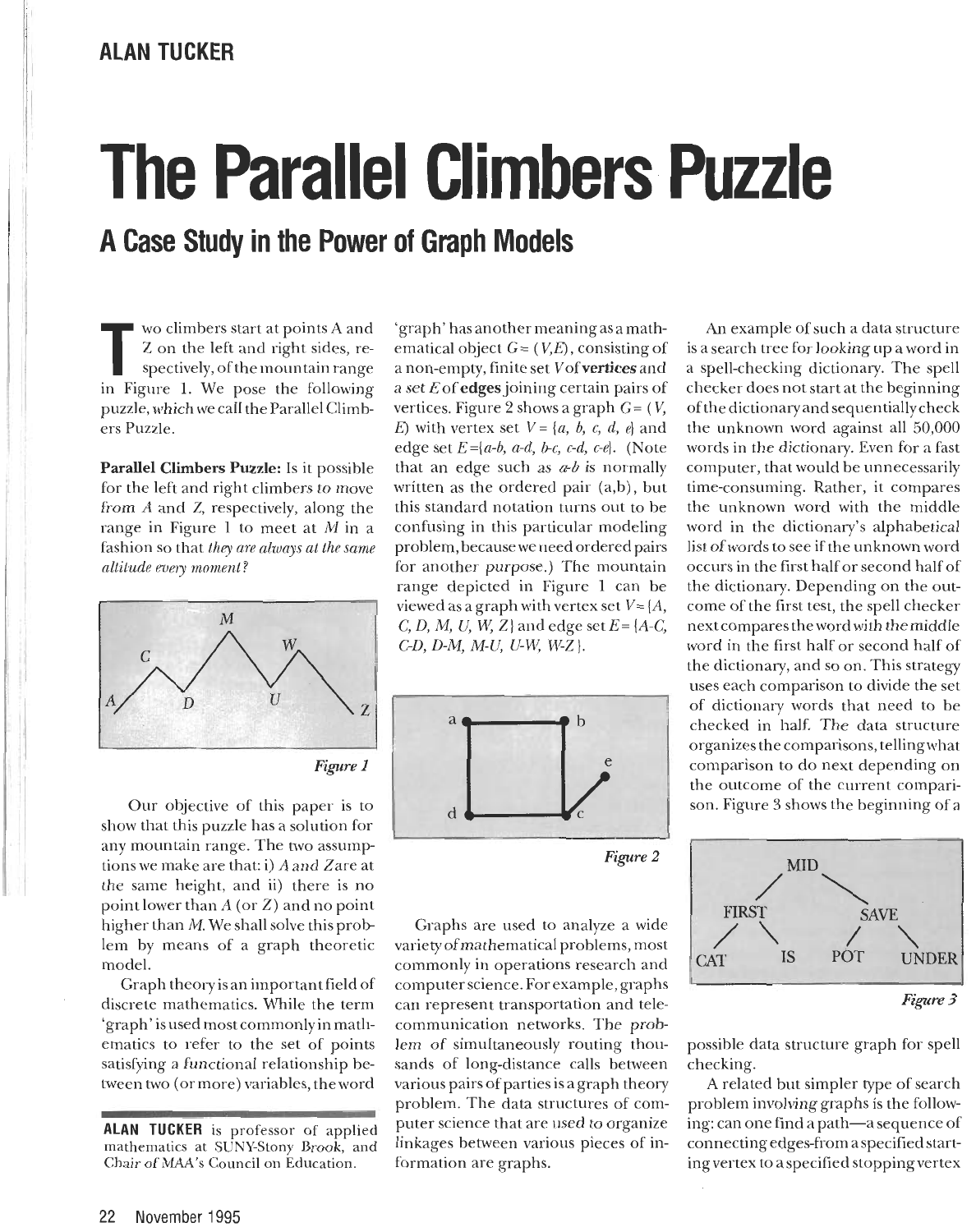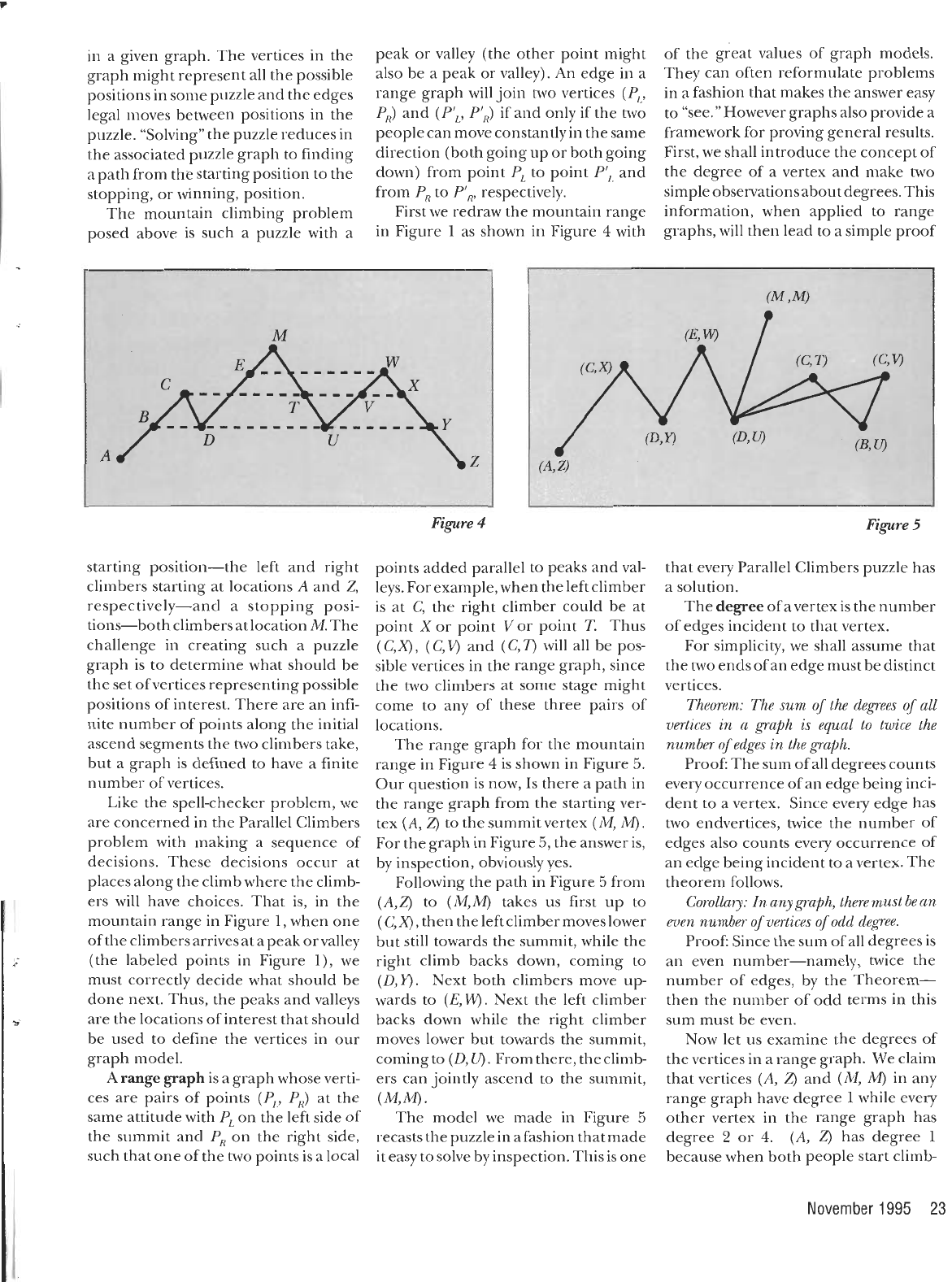This problem was first posed by James Whittaker in 1966 (you can ch...
In mathematical terms the problem can be re-written as: Let $s(\... When one or two of the climbers hit a critical point (peak or valle... The sum of all the degrees of a graph$D_{Total}\$ is composed by th...
In this paper we prove that the parallel climbers problem has a sol...